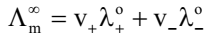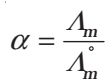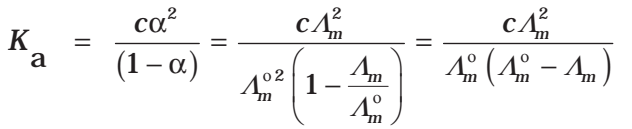## Kohlrausch’s Law

It states that the limiting molar conductivity of an electrolyte can be represented as the sum of the individual contributions of the anion and cation of the electrolyte. In general, if an electrolyte of dissociation gives v+ cations and v– anions then its limiting molar conductivity is given byHere, λo+ and λo are the limiting molar conductivities of cations and anions respectively.

## Application of Kohlrausch’s Law

• Calculation of molar conductivities of the weak electrolyte at infinite dilution:

For example, the molar conductivity of acetic acid at infinite dilution can be obtained from the knowledge of molar conductivities at infinite dilution of the strong electrolyte like HCl, CH3COONa and NaCl as illustrated below.• Determination of Degree of Dissociation of Weak Electrolytes• Determination of Dissociation constant (K) of Weak Electrolytes